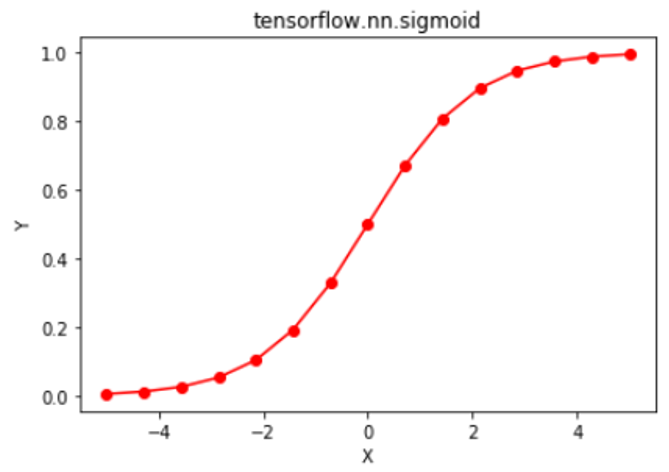# Python | Tensorflow nn.sigmoid()

Tensorflow is an open-source machine learning library developed by Google. One of its applications is to develop deep neural networks.

The module `tensorflow.nn` provides support for many basic neural network operations.

One of the many activation functions is the sigmoid function which is defined as.

Sigmoid function outputs in the range (0, 1), it makes it ideal for binary classification problems where we need to find the probability of the data belonging to a particular class. The sigmoid function is differentiable at every point and its derivative comes out to be. Since the expression involves the sigmoid function, its value can be reused to make the backward propagation faster.

Sigmoid function suffers from the problem of “vanishing gradients” as it flattens out at both ends, resulting in very small changes in the weights during backpropagation. This can make the neural network refuse to learn and get stuck. Due to this reason, usage of the sigmoid function is being replaced by other non-linear functions such as Rectified Linear Unit (ReLU).

The function `tf.nn.sigmoid()` [alias `tf.sigmoid`] provides support for the sigmoid function in Tensorflow.

Syntax: tf.nn.sigmoid(x, name=None) or tf.sigmoid(x, name=None)

Parameters:
x: A tensor of any of the following types: float16, float32, float64, complex64, or complex128.
name (optional): The name for the operation.

Return type: A tensor with the same type as that of x.

Code #1:

 `# Importing the Tensorflow library ` `import` `tensorflow as tf ` ` `  `# A constant vector of size 6 ` `a ``=` `tf.constant([``1.0``, ``-``0.5``, ``3.4``, ``-``2.1``, ``0.0``, ``-``6.5``], dtype ``=` `tf.float32) ` ` `  `# Applying the sigmoid function and ` `# storing the result in 'b' ` `b ``=` `tf.nn.sigmoid(a, name ``=``'sigmoid'``) ` ` `  `# Initiating a Tensorflow session ` `with tf.Session() as sess: ` `    ``print``(``'Input type:'``, a) ` `    ``print``(``'Input:'``, sess.run(a)) ` `    ``print``(``'Return type:'``, b) ` `    ``print``(``'Output:'``, sess.run(b)) `

Output:

```Input type: Tensor("Const_1:0", shape=(6, ), dtype=float32)
Input: [ 1.        -0.5        3.4000001 -2.0999999  0.        -6.5      ]
Return type: Tensor("sigmoid:0", shape=(6, ), dtype=float32)
Output: [ 0.7310586   0.37754068  0.96770459  0.10909683  0.5         0.00150118]
```

Code #2: Visualization

 `# Importing the Tensorflow library ` `import` `tensorflow as tf ` ` `  `# Importing the NumPy library ` `import` `numpy as np ` ` `  `# Importing the matplotlib.pylot function ` `import` `matplotlib.pyplot as plt ` ` `  `# A vector of size 15 with values from -5 to 5 ` `a ``=` `np.linspace(``-``5``, ``5``, ``15``) ` ` `  `# Applying the sigmoid function and ` `# storing the result in 'b' ` `b ``=` `tf.nn.sigmoid(a, name ``=``'sigmoid'``) ` ` `  `# Initiating a Tensorflow session ` `with tf.Session() as sess: ` `    ``print``(``'Input:'``, a) ` `    ``print``(``'Output:'``, sess.run(b)) ` `    ``plt.plot(a, sess.run(b), color ``=` `'red'``, marker ``=` `"o"``)  ` `    ``plt.title(``"tensorflow.nn.sigmoid"``)  ` `    ``plt.xlabel(``"X"``)  ` `    ``plt.ylabel(``"Y"``)  ` ` `  `    ``plt.show() `

Output:

```Input: Input: [-5.         -4.28571429 -3.57142857 -2.85714286 -2.14285714 -1.42857143
-0.71428571  0.          0.71428571  1.42857143  2.14285714  2.85714286
3.57142857  4.28571429  5.        ]
Output: [ 0.00669285  0.01357692  0.02734679  0.05431327  0.10500059  0.19332137
0.32865255  0.5         0.67134745  0.80667863  0.89499941  0.94568673
0.97265321  0.98642308  0.99330715]
```My Personal Notes arrow_drop_upCheck out this Author's contributed articles.

If you like GeeksforGeeks and would like to contribute, you can also write an article using contribute.geeksforgeeks.org or mail your article to contribute@geeksforgeeks.org. See your article appearing on the GeeksforGeeks main page and help other Geeks.

Please Improve this article if you find anything incorrect by clicking on the "Improve Article" button below.

Improved By : sanskar27jain